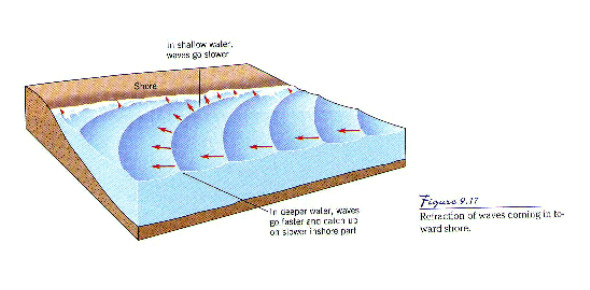# Currents, Waves, And Tides

15 Questions | Total Attempts: 7474SettingsTest over currents, waves, and tides aimed at sixth grade earth science students.

• 1.
The wind blows on the water, passing it's energy to the water. As this energy passes through the water, in what way do the water molecules move?
• A.

In waves towards the shore

• B.

In a small vertical circle

• C.

In the direction of the waves

• D.

Against the currents

• 2.
The lowest point of a wave is the:
• A.

Crest

• B.

Wavelength

• C.

Trough

• D.

Frequency

• 3.
The highest point of a wave is:
• A.

Crest

• B.

Wavelength

• C.

Trough

• D.

Frequency

• 4.
When the wind blows across a body of water (like the ocean), ________________ between the water and air causes the water to move.
• A.

Ions

• B.

Gravity

• C.

Friction

• D.

Density

• 5.
The size of a wave is NOT affected by the:
• A.

Length and time the wind blows across the water

• B.

Salinity of the water

• C.

Strength of the wind (how hard the wind is blowing)

• D.

Distance the wind blows across the water

• 6.
This occurs when circulation in the ocean brings deep, cold water to the ocean surface:
• A.

Rotation of the earth

• B.

Density

• C.

Coriolis effect

• D.

Upwelling

• 7.
______________ water rises and ________________ water sinks
• A.

Warm, cold

• B.

Cold, warm

• C.

None of the above

• D.

All of the above

• 8.
The amount of salt in a given amount of water is:
• A.

Coriolis effect

• B.

Salinity

• C.

Density current

• D.

Surface current

• 9.
What causes the currents to curve instead of moving in straight lines?
• A.

The moon's gravity

• B.

Wave height

• C.

Coriolis effect

• D.

Density currents

• 10.
What is the main cause of the gulf steam?
• A.

Storms

• B.

Winds that blow now and then

• C.

Winds that blow in regular directions all the time

• D.

The moon's gravity

• 11.
Density currents are caused by:
• A.

Differences in temperature

• B.

Differences in salinity

• C.

The moon's gravity

• D.

A and B are both correct

• 12.
What type of ocean motion is influenced by the moon and the sun?
• A.

Density currents

• B.

Surface currents

• C.

Waves

• D.

Tides

• 13.
Surface currents are caused by
• A.

Wind that blows every now and then

• B.

Wind that blows in regular directions

• C.

The moon's gravitational pull

• D.

Differences in salinity and temperature

• 14.
When the sun, moon, and earth are all aligned (in a straight line), you have a
• A.

Density current

• B.

Neap tide

• C.

Surface current

• D.

Spring tide

• 15.
The sun's rays are strongest at the____________ and weakest at the _______________:Hint: the stronger the rays, the hotter it gets!!!
• A.

Equator, poles

• B.

Poles, equator

• C.

Ocean floor, ocean surface

• D.

Midocean ridge, continental shelf

Related TopicsBack to top